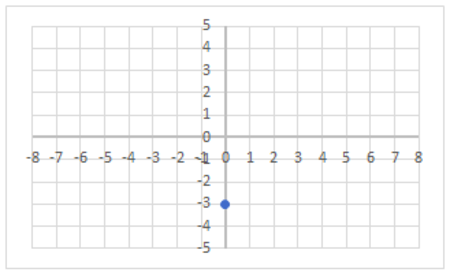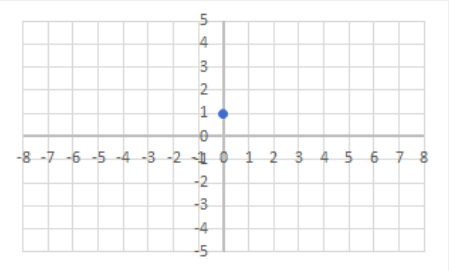Back
Plot a Line by Connecting Points
> ... Math > Slopes, Intercepts, Equation of a Straight Line > Plot a Line by Connecting Points

One method for graphing a function is to find two or more points on the line, plot them, and then connect those dots with a line. To find points on the line, choose any value for x, then plug that number in the function and solve for the unknown variable y. (If you need help on this topic, please review the mini lesson: "Substitute values into an equation and Solve for a variable.")

The following video will show an example of how to do this. In this example, we find four points. It is only necessary to do two points, but doing more will help you check for mistakes.

Plotting a Line in Slope-Intercept Form

Not all equations come in Slope-Intercept form, but we can still graph them. The following video will show how to graph an equation in Standard Form, where all of the variables are on the same side of the equation. It’s still a straight line. Similar to the previous video, we find two or more points to plot, then connect them to form the line.

Graphing any Linear Equation (straight line)

### Practice Problems

1. Match the equation of the line with its graph.
a. $${\text{y}}=4{\text{x}}-3$$ (
Solution
Solution:
Graph #2
)
b. $${\text{y}}=-2{\text{x}}+3$$ (
Solution
Solution:
Graph #1
)
c. $${\text{y}}= \dfrac{1}{3}{\text{x}}$$ (
Solution
Solution:
Graph #3
Details:
The equation, $$y={\color{Red}4}x−{\color{Blue}3}$$, is written in slope-intercept form, which is $$y = {\color{Red}m}x + {\color{Blue}b}$$, where m is the slope and b is the y-intercept. We need to find the graph that has a $${\color{Red}slope}$$ of $${\color{Red}4}$$ and a $${\color{Blue}y-intercept}$$ of $${\color{Blue}-3}$$.

First, we look at Graph #1. We see that the y-intercept is 3 and the slope is negative. It is not the correct graph.Then we look at Graph #2. We see that the y-intercept is $$−3$$ and the slope is positive. The rise is 4 and the run is 1, so the slope is $$m=\dfrac{4}{1}$$, which is equal to 4. This is the correct graph.We can also check Graph #3. We see that the y-intercept is 0 and the slope is positive. The rise is 1 and the run is 3 so the slope is $$m=\dfrac{1}{3}$$.This is not the correct graph.)
1.2.3.2. Create a graph of the line represented by the equation. (
Solution
Solution:
Note: Your line may look different than this one, but the x-intercept and the y-intercept should be the same.)
$${\text{y}}=2{\text{x}}-5$$
3. Create a graph of the line represented by the equation. (
Solution
Solution:
Note: Your line may look different than this one, but the x-intercept and the y-intercept should be the same.)
$${\text{y}}=\dfrac{-1}{2} {\text{x}}+2$$
4. Graph the line represented by the equation. (
Solution
Solution:
Note: Your line may look different than this one, but the x-intercept and the y-intercept should be the same.)
$$3{\text{x}}+4{\text{y}}=-12$$
5. Graph the line represented by the equation. (
Solution
Solution:
Note: Your line may look different than this one, but the x-intercept and the y-intercept should be the same.Details:
To graph the line, $$\dfrac{1}{3}{\text{x}}{-}{\dfrac{1}{2}}{\text{y}}=5$$ , we need to find at least two points that are on the line. We’ll start with a table of values:

x y
- -
- -

To find the y-intercept, we’ll plug in 0 for x and find the corresponding y-value:

$${\dfrac{1}{3}(0)}{-}{\dfrac{1}{2}}{\text{y}}=5$$

We know that $$\dfrac{1}{3}\times0=0$$ so our equation now reads:

$$0-\dfrac{1}{2}{\text{y}}=5$$

Which equals:

$$-\dfrac{1}{2}{\text{y}}=5$$

Next, we multiply both sides by $$-\dfrac{2}{1}$$ to cancel out the $$-\dfrac{1}{2}$$ and solve for y. We also write 5 as $$\frac{5}{1}$$ to make solving easier:

$$\left(-\dfrac{2}{1}\right)\left(-\dfrac{1}{2}{\text{y}}\right)=\dfrac{5}{1}\left(-\dfrac{2}{1}\right)$$

On the left side we multiply $$\left(-\dfrac{2}{1}\right)\times\left(-\dfrac{1}{2}\right)$$ together which equals 1. We now have:

$$1{\text{y}}=\dfrac{5}{1}\left(-\dfrac{2}{1}\right)$$

To multiply $$\dfrac{5}{1}\times\left(-\dfrac{2}{1}\right)$$ , we multiply straight across which equals $$-\frac{10}{1}$$.

$$1{\text{y}}= -\dfrac{10}{1}$$

Which simplifies to:

$$1{\text{y}}=-10$$

So the point $$(0,−10)$$ is on the line and we can update our table accordingly.

x y
0 -10
- -

To find the x-intercept, we follow the same process plugging in 0 for y and then finding the corresponding x-value:

$$\dfrac{1}{3}{\text{x}}{-}{\dfrac{1}{2}}\left(0\right)=5$$

Which equals:

$$\dfrac{1}{3}{\text{x}}{-}0=5$$

Which equals:

$$\frac{1}{3}{\text{x}}=5$$

Then multiply both sides by 3:

$$(3)(\dfrac{1}{3}){\text{x}}=5(3)$$

Which gives us:

$$(\cancel{3})(\dfrac{1}{\cancel{3}}){\text{x}}=5(3)$$

and

$${\text{x}}=15$$

Now we have an additional point, (15,0).

x y
0 -10
15 0

Using the points $$(15,0)$$ and $$(0,−10)$$ we can graph the line. First, we graph the points:Then we draw a line through both points:)
$$\dfrac{1}{3}{\text{x}}{-}{\dfrac{1}{2}}{\text{y}}=5$$
6. Plot the y-intercept for the line: (
Solution
Solution:Details:
The equation of our line is $$y=−3x+{\color{Red}2}$$ and it is written in slope-intercept form, which is $$y=mx + {\color{Red}b}$$, where m is the slope and $${\color{Red}b}$$ is the $${\color{Red}y-intercept}$$. So the y-intercept is (0,2)
)
$${\text{y}}=-3{\text{x}}+2$$
7. Use the information in the previous question to answer this problem. (
Solution
Solution:
Note: Answers will vary depending on what value of x you choose. Several possible points are shown. All correct points will lie upon the line, but since the line is infinite we cannot show the entire line or all possible points on it.Details:
We want to graph this line and already have the y-intercept which is (0, 2). We need one more point to graph the line. We’ll choose $${\color{Red}x = 1}$$ and find the corresponding y-value (you can choose any value for x. We chose x = 1 for convenience). Our equation is:

$$y=−3{\color{Red}x}+2$$

Replace x with $${\color{Red}1}$$:

$$y=−3{\color{Red}(1)}+2$$

Then simplify:

$$y=−{\color{Red}3}+2$$

$$y=−1$$

So another point on our line is $$(1,−1)$$. Now we can graph both points:Then draw a line through both points to graph the line:)
Now, choose an x-value (besides $$x=0$$) and plot the point on the line $$y=−3x+2$$ that corresponds to this value. Then, use these two points to graph the line.
8. Plot the y-intercept for the line (
Solution
Solution:)
$${\text{y}} = \dfrac{1}{2}{\text{x}} - 3$$
9. Use the information in the previous question to answer this problem. (
Solution
Solution:
Note: Answers will vary depending on what value of x you choose. Several possible points are shown. All correct points will lie upon the line, but since the line is infinite we cannot show the entire line or all possible points on it.)
Now, choose an x-value (besides $$x=0$$) and plot the point on the line $${\text{y}} = \dfrac{1}{2}{\text{x}} - 3$$ that corresponds to this value. Then, use these two points to graph the line.
10. Plot the y-intercept for the line: (
Solution
Solution:)
$${\text{y}} = -\dfrac{3}{5}{\text{x}} + 1$$
11. Use the information in the previous question to answer this problem. (
Solution
Solution:
Note: Answers will vary depending on what value of x you choose. Several possible points are shown. All correct points will lie upon the line, but since the line is infinite we cannot show the entire line or all possible points on it.)
Now, choose an x-value (besides $$x=0$$) and plot the point on the line $${\text{y}} = -\dfrac{3}{5}{\text{x}} + 1$$ that corresponds to this value. Then, use these two points to graph the line.

## Need More Help?

1. Study other Math Lessons in the Resource Center.
2. Visit the Online Tutoring Resources in the Resource Center.